﻿ Modulus
Liquid Studio Documentation
Data Mapping / Data Mapper Functions / By Category / Maths / Modulus
In This Topic
Modulus
In This Topic

Modulus
Maths
##### Icon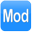##### Description
Return modulus (remainder) of a division calculation
##### Inputs
 Dividend Value to divide Divisor Value to divide by
##### Outputs
 Result Remainder after dividing first input by second
##### Properties
 None -

##### Usage

The Data Mapper Maths Modulus function allows you to calculate remainders after division on input values. You can use the Modulus function with input source data or in conjunction with other arithmetic functions in the Maths section. To apply the Maths Modulus function, use the following process:

Create a new Data Mapper file, dragging your XML data source and targets into the editor area. For this example we are using bookclub_source.xsd, an XML Schema Definition inferred from the following source:

Source XML
Copy Code
```<bookclub>
<group>
<group_number>12</group_number>
<books>57</books>
<members>17</members>
</group>
<group>
<group_number>10</group_number>
<books>43</books>
<members>12</members>
</group>
<group>
<group_number>5</group_number>
<books>79</books>
<members>30</members>
</group>
</bookclub>
```

For our target schema we will be using bookclub_target.xsd, inferred from the following XML:

Target XML
Copy Code
```<club>
<section ref="14" joined="27">
<volumes_each>4</volumes_each>
<volumes_left>7</volumes_left>
</section>
<section ref="7" joined="35">
<volumes_each>2</volumes_each>
<volumes_left>19</volumes_left>
</section>
<section ref="15" joined="18">
<volumes_each>5</volumes_each>
<volumes_left>2</volumes_left>
</section>
</club>
```

The data models the members and books for a book club. Each group within the club has a certain number of books to share between the members. As you can see from the target data, as well as mapping the source data values, we need to include an indicator of how many books we can allocate to each member, plus a figure representing how many are left over - we will use the modulus to calculate this. Here is the Data Mapper with Source and Target imported:Drag the Maths Modulus function from the Component Palette into the mapping area: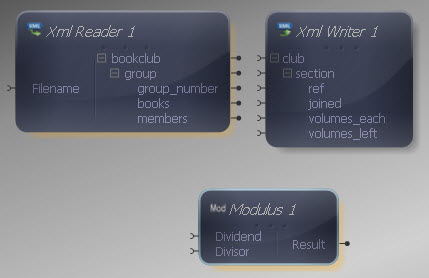The Modulus component has two inputs, representing the value to divide and the value to divide it with. The output represents the remainder following this division operation. Both inputs and output of the Modulus function may be connected to items in the XML Reader/ Writer or other components in the Mapper.

Connect the Dividend input in the Modulus function to the "books" output in the XML Reader and the divisor input to the "members" output.Now connect the Modulus output to the "volumes_left" input in the XML Reader.That's the remainder part of the calculation completed, but we also need to indicate how many books there are per person, so drag a Divide function onto the Mapper. As with the Modulus function, connect the Dividend input to the "books" output in the XML Reader and the Divisor input to the "members" output.Now, we need the number of books per person to be a whole number, so let's cast the division result to an integer. Drag a Cast function from the Type section onto the Mapper. Right-click it and choose Show Properties. Choose an integer type from the drop-down list.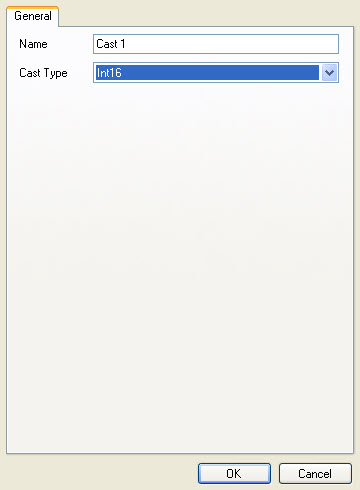Now connect the Cast input to the Divide function output.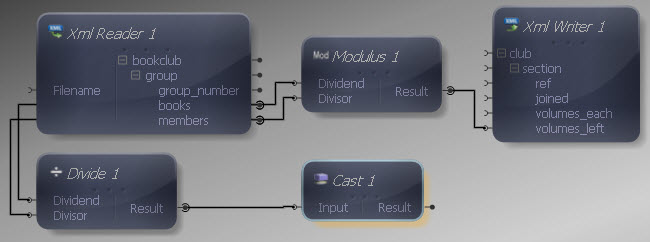Now we can connect the Cast output to the "volumes_each" input in the XML Writer.Finally let's make the remaining input and output connections. Remember to map "members" to "joined" - although we have used the members value for our division and modulus calculations, we have not yet mapped them to the output.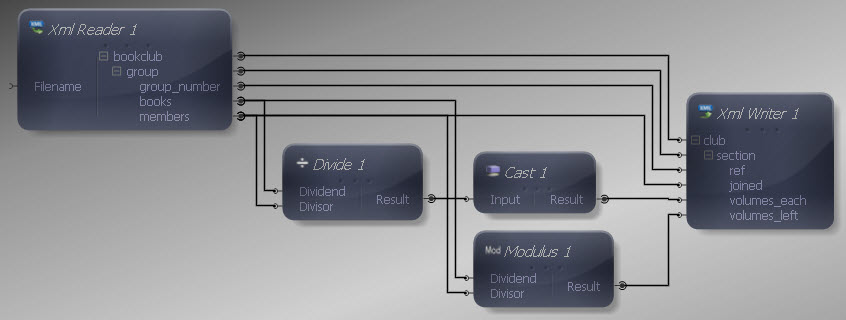We can now execute the transform by pressing Shift-F5 or the Execute button (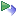). The transform is applied and the file we selected as output opens in the editor:

Output XML
Copy Code
```<club>
<section ref="12" joined="17">
<volumes_each>3</volumes_each>
<volumes_left>6</volumes_left>
</section>
<section ref="10" joined="12">
<volumes_each>3</volumes_each>
<volumes_left>7</volumes_left>
</section>
<section ref="5" joined="30">
<volumes_each>2</volumes_each>
<volumes_left>19</volumes_left>
</section>
</club>
```

The output contains an indicator of how many books can be allocated to each member in a group / section as well as how many are left over in each case.

This is a scalar function so the standard rules apply if multiple values are presented to one or more inputs.